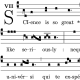## Volume forms on the mass-shell

The setting for dynamics is the cotangent bundle$\mathcal{T}^*\mathcal{M}$ of a manifold$\mathcal{M}$ with pseudo-Riemannian metric$g_{\mu\nu}$; relevant observables can be functions of both position and momentum. For example, the distribution function$f(x,p)$, which is the number density of particles in phase space ($x^\mu$ and$p^\mu$ are coordinates on$\mathcal{M}$ and$\mathcal{T}^*_x\mathcal{M}$ respectively).

The spatial volume form (integral measure) is$\mathrm{vol}(\mathcal{N}) \equiv \sqrt{g(x)} dx^1 \wedge \ldots \wedge dx^n$, where$g(x)$ is the determinant of the metric evaluated at$x \in M$; and the volume form for the full phase space is$dx^1 \wedge \ldots \wedge dx^n \wedge dp_1 \wedge \ldots \wedge dp_n$. If we want to integrate out the momentum-dependence of some observable, we need just the momentum-part of the volume form. From the two expressions above we can see that this is$\mathrm{vol}(\mathcal{T}^*_x\mathcal{M}) = \frac{1}{\sqrt{g(x)}} dp^1 \wedge \ldots \wedge dp^n$.

However, paths that obey the classical equations of motion are constrained to lie on the mass-shell: in flat Lorentzian spacetime this is a hyperboloid in the momentum cotangent space$\left(\mathbb{R}^{1,3}\right)^*$, given by$-p_0^2 + p_i^2 = -m^2$, where$p_0$ is the energy,$p_i$ the spatial 3-momentum and m the rest mass of a particular particle. However, in fully general curved spacetime the corresponding condition gives a hypersurface$\mathcal{N} \equiv \{(x,p) \in \mathcal{T}^*\mathcal{M} \: | \: p^\mu p_\mu = -m^2 \}$.

Because the permitted region of phase space has been restricted, we can eliminate one momentum component from the integral, treating it as a function of the other coordinates. Conventionally we pick$p_0$ to be this unwanted component, writing$p_0 = p_0(p_i)$, and note that the equation for$\mathcal{N}$ can be written$p_\mu p^\mu = p_0 p^0 + p_i p^i = -m^2$, which allows us to solve for$p_0$.

We then find the volume form induced on$\mathcal{N}$. Let$n$ be the unit vector field normal to$\mathcal{N}$:$n = \left.\frac{d(p_\mu p^\mu)}{\left\|d(p_\mu p^\mu)\right\|}\right|_\mathcal{N}$

The denominator works out to be$-4m^2$. Noting that the metric is a function of$x$ alone, we perform the derivative on the numerator and so find that$n = \frac{2p_\mu dp^\mu}{-4m^2} = \frac{-p_\mu dp^\mu}{2m^2}$

(the normal index convention for vectors and covectors is swapped round, as we are working on$\mathcal{T}^*\mathcal{M}$, so the coordinates$p_\mu$ are covariant to begin with).

In general, the volume form induced from a manifold$\mathcal{M}$ onto a submanifold$\mathcal{N}$ with normal VF$n$ is$\mathrm{vol}(\mathcal{N}) = \left. n \:\lrcorner\: \mathrm{vol}(\mathcal{M}) \right|_\mathcal{N}.$

For the present purposes we therefore have$\sqrt{g(x)} \mathrm{vol}(\mathcal{N}) = n \:\lrcorner\: \left( dp_0 \wedge dp_1 \wedge dp_2 \wedge dp_3 \right) \\ \:\: = (n \:\lrcorner\: dp_0) dp_1 \wedge dp_2 \wedge dp_3 - (n \:\lrcorner\: dp_1) dp_0 \wedge dp_2 \wedge dp_3 \\ \:\:\:\:\:\: + (n \:\lrcorner\: dp_2) dp_0 \wedge dp_1 \wedge dp_3 - (n \:\lrcorner\: dp_3) dp_0 \wedge dp_1 \wedge dp_2$

We differentiate the condition$p^\mu p_\mu = -m^2$ with respect to a spatial component$p_i$ and rearrange, giving (note the positions of the indices):$\frac{\partial p_0}{\partial p_i} = \frac{-p^i}{p^0} \\ dp_0 = \frac{\partial p_0}{\partial p_i}dp_i = \frac{-p^i}{p^0}dp_i.$

Expressions of the form$dp_0 \wedge dp_i \wedge dp_j$ can be simplified to involve only the$k$-component of$dp_0$ ($i \neq k \neq j$ ), as repetition of a form in a wedge product sets the entire expression to zero. We also have$n \:\lrcorner\: dp_\mu = n_\mu = \frac{-p_\mu}{2m^2}$.

So, putting it all together,$\sqrt{g(x)} \mathrm{vol}(\mathcal{N}) = \\ \:\: \left( \frac{-p_0}{2m^2} \right) dp_1 \wedge dp_2 \wedge dp_3 - \left( \frac{-p_1}{2m^2} \frac{-p^1}{p^0} \right) dp_1 \wedge dp_2 \wedge dp_3 \\ \:\:\:\:\:\: + \left( \frac{-p_2}{2m^2} \frac{-p^2}{p^0} \right) dp_2 \wedge dp_1 \wedge dp_3 - \left( \frac{-p_3}{2m^2} \frac{-p^3}{p^0} \right) dp_3 \wedge dp_1 \wedge dp_2 \\ \:\: = \frac{-1}{2 m^2 p^0}\left(p_0p^0 + p_ip^i\right) dp_1 \wedge dp_2 \wedge dp_3 \\ \:\: = \frac{-(-m^2)}{2 m^2 p^0} dp_1 \wedge dp_2 \wedge dp_3$

And so the final result is$\mathrm{vol}(\mathcal{N}) = \frac{dp_1 \wedge dp_2 \wedge dp_3}{2 p^0 \sqrt{g(x)}} \\$

which has the expected form. Integrals over momentum space therefore look like$\int_\mathcal{N} f(p_i) \frac{dp_1 \wedge dp_2 \wedge dp_3}{2 E(p_i) \sqrt{g(x)}}.$

Everything we wrote down was manifestly covariant, so this volume form transforms in the correct way under general coordinate transformations. The rest mass$m$ does not appear in the final volume form, so we are free to set$m = 0$ if we choose, as is the case with photons.